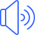weigh

المضارع او المصدرweighed

الماضيweighed

التصريف الثالثweighing

صيغة الـ ing

# استخدامات الفعل weigh مع جميع الازمنة الانجليزية

Simple Present Tense

# زمن المضارع البسيط

I weigh
You weigh
He weighs
She weighs
It weighs
We weigh
You weigh
They weigh

لا يفوتك تحميل تطبيقنا المجاني الشامل والذي سوف يغنيك عن كل شي حيث سوف يبدء معك من مستوى الاحرف الانجليزية ويستمر معك الى مستوى الاحتراف بأذن الله مع اختبارات تفاعلية متعددة وشرح مفصل لكل صغيرة وكبيرة علما ان جميع الدروس والمقاطع الصوتية تعمل بدون الحاجة الى الانترنت. ولاتنسى تقيم التطبيق اذا كنت بالفعل حملته على جهازك

تطبيق الايفون تطبيق الاندرويد

Simple Past Tense

# زمن الماضي البسيط

I weighed
You weighed
He weighed
She weighed
It weighed
We weighed
You weighed
They weighed

Simple Future Tense

# زمن المستقبل البسيط

I will weigh
You will weigh
He will weigh
She will weigh
It will weigh
We will weigh
You will weigh
They will weigh

Present Perfect Tense

# زمن المضارع التام

I have weighed
You have weighed
He has weighed
She has weighed
It has weighed
We have weighed
You have weighed
They have weighed

Past Perfect Tense

# زمن الماضي التام

Future Perfect Tense

# زمن المستقبل التام

I will have weighed
You will have weighed
He will have weighed
She will have weighed
It will have weighed
We will have weighed
You will have weighed
They will have weighed

Present Continuous Tense

# زمن المضارع المستمر

I am weighing
You are weighing
He is weighing
She is weighing
It is weighing
We are weighing
You are weighing
They are weighing

Past Continuous Tense

# زمن الماضي المستمر

I was weighing
You were weighing
He was weighing
She was weighing
It was weighing
We were weighing
You were weighing
They were weighing

Future Continuous Tense

# زمن المستقبل المستمر

I will be weighing
You will be weighing
He will be weighing
She will be weighing
It will be weighing
We will be weighing
You will be weighing
They will be weighing

Present Perfect Continuous Tense

# زمن المضارع التام المستمر

I have been weighing
You have been weighing
He has been weighing
She has been weighing
It has been weighing
We have been weighing
You have been weighing
They have been weighing

Past Perfect Continuous Tense

# زمن الماضي التام المستمر

Future Perfect Continuous Tense

# زمن المستقبل التام المستمر

I will have been weighing
You will have been weighing
He will have been weighing
She will have been weighing
It will have been weighing
We will have been weighing
You will have been weighing
They will have been weighing

لكي تتابع دروسنا الجديدة بالصوت والصورة والشرح المفصل انصحك بالاشتراك في قناتنا على اليوتيوب

او انشر الصفحة على مواقع التواصل الاجتماعي

### كلمات دالة

weigh تصريف فعل

weighed تصريف الفعل

weighed تصريف فعل

weigh تصريف الفعل

weigh ترجمة و معنى كلمة

weigh معنى الفعل

weigh ترجمة الفعل